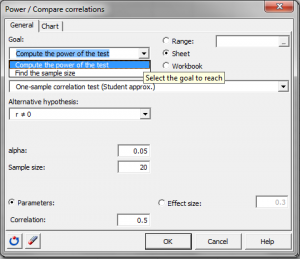# Statistical Power for comparing correlations

Ensure optimal power or sample size using power analysis. Power for the comparison of correlations available in Excel using the XLSTAT statistical software.## Statistical Power of correlation comparison tests

XLSTAT offers a test to compare correlations. XLSTAT can calculate the power or the number of observations necessary for this test. When testing a hypothesis using a statistical test, there are several decisions to take:

• The null hypothesis H0 and the alternative hypothesis Ha.
• The statistical test to use.
• The type I error also known as alpha. It occurs when one rejects the null hypothesis when it is true. It is set a priori for each test and is 5%.

The type II error or beta is less studied but is of great importance. In fact, it represents the probability that one does not reject the null hypothesis when it is false. We cannot fix it up front, but based on other parameters of the model we can try to minimize it. The power of a test is calculated as 1-beta and represents the probability that we reject the null hypothesis when it is false.

We therefore wish to maximize the power of the test. XLSTAT calculates the power (and beta) when other parameters are known. For a given power, it also allows to calculate the sample size that is necessary to reach that power.

The statistical power calculations are usually done before the experiment is conducted. The main application of power calculations is to estimate the number of observations necessary to properly conduct an experiment. XLSTAT allows you to compare:

• One correlation to 0.
• One correlation to a constant.
• Two correlations.

## Calculations for the Statistical Power of tests comparing correlations

The power of a test is usually obtained by using the associated non-central distribution. For this specific case we will use an approximation in order to compute the power.

### Statistical Power for comparing one correlation to 0

The alternative hypothesis in this case is: H a: r ≠ 0 The method used is an exact method based on the non-central Student distribution. The non-centrality parameter used is the following: NCP = √ r²/(1-r²)* √N The part r²/(1-r²) is called effect size.

### Statistical Power for comparing one correlation to a constant

The alternative hypothesis in this case is: Ha: r ≠ r0. The power calculation is done using an approximation by the normal distribution. We use the Fisher Z-transformation: Zr = ½ log[(1+r)/(1-r)]. The effect size is: Q = |Zr - Zr0|. The power is then found using the area under the curve of the normal distribution to the left of Zp: Zp = Q * √N - 3 - Zreq where Zreq is the quantile of the normal distribution for alpha.

### Statistical Power for comparing two correlations

The alternative hypothesis in this case is: Ha: r1 – r2 ≠ 0. The power calculation is done using an approximation by the normal distribution. We use the Fisher Z-transformation: Zr = ½ log[(1+r)/(1-r)]. The effect size is: Q = |Zr1 - Zr2|. The power is then found using the area under the curve of the normal distribution to the left of Zp: Zp = Q * √(N’ – 3)/2 - Zreq where Zreq is the quantile of the normal distribution for alpha and N’ = [2*(N1 - 3)*(N2 - 3)]/[N1 + N2 - 6] + 3.

### Calculating sample size for a correlation comparison test

To calculate the number of observations required, XLSTAT uses an algorithm that searches for the root of a function. It is called the Van Wijngaarden-Dekker-Brent algorithm (Brent, 1973). This algorithm is adapted to the case where the derivatives of the function are not known. It tries to find the root of:

power (N) - expected_power

We then obtain the size N such that the test has a power as close as possible to the desired power.

## Effect size for correlation comparison tests

This concept is very important in power calculations. Indeed, Cohen (1988) developed this concept. The effect size is a quantity that will allow to calculate the power of a test without entering any parameters but will tell if the effect to be tested is weak or strong. In the context of comparisons of correlations conventions of magnitude of the effect size are:

• Q=0.1, the effect is small.
• Q=0.3, the effect is moderate.
• Q=0.5, the effect is strong.

XLSTAT allows you to enter directly the effect size.### analyze your data with xlstat

14-day free trial

Included in

Related features